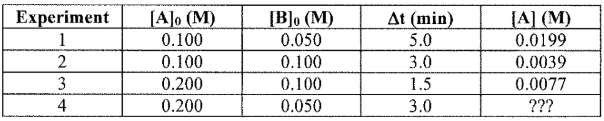# Problem: The following reaction is studied at 25 °C in benzene solution:CH3OH + (C6H5)3CCl → CH3OC(C6H5)3 + HCl   A                    BThe following experimental data have been collected:What is the rate law that is consistent with the above data? Calculate the value of the rate constant, k, including units.

###### FREE Expert Solution

We’re being asked to calculate the value of the rate constant, k, including units.

CH3OH + (C6H5)3CCl → CH3OC(C6H5)3 + HCl

A                 B

Recall that the rate law only focuses on the reactant concentrations and has a general form of:

k = rate constant
A & B = reactants
x & y = reactant orders

We’re going to calculate the rate law and the rate constant using the following steps:

97% (393 ratings)###### Problem Details

The following reaction is studied at 25 °C in benzene solution:

CH3OH + (C6H5)3CCl → CH3OC(C6H5)3 + HCl

A                    B

The following experimental data have been collected:What is the rate law that is consistent with the above data? Calculate the value of the rate constant, k, including units.